## Tubing Length Change due to Ballooning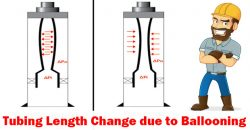Ballooning is a change in average pressure which causes a radial contraction or swelling. If the tubing is free to move, the length of tubing will be either longer or shorter. If tubing cannot be moved, stress will be created in the tubing body.

Figure 1 illustrates ballooning of tubing. This will happen when internal pressure is higher than external pressure. Tubing will shorten if the tubing is free to move. If the tubing cannot be moved, ballooning will create a tension force on the packer.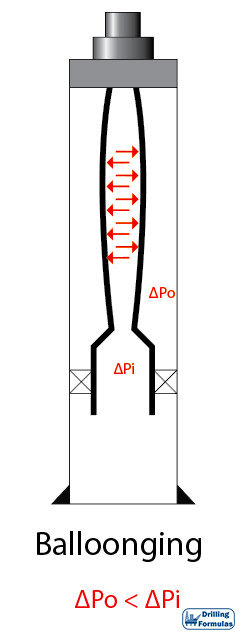Figure 1 – Ballooning

## Tubing Length Change due to Piston Effect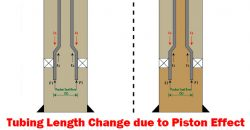This article demonstrates a piston effect and how it will change the length of tubing with full detailed calculations.

For the analysis, Lubinski’s sign conventions are used.

Compression force = ⇑(+)

Tensile force = ⇓ (-)

Shorten in Length = (-)

Elongate in length = (+)

Note: The tubing is free to move.

Please see this in the reference section to get a full paper.

Total force change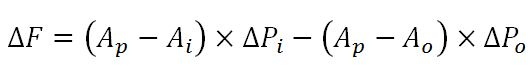Total length change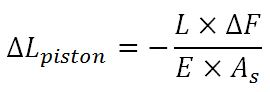Continue reading

## Piston Effect on Tapered String and Expansion Device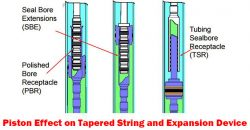In many situations, a completion string may consist of several size of strings and components so total axial force must be carefully considered by applying a free body diagram concept. When the tubing string is free to move, forces will affect the tubing above where the force is applied.

Tapered String

Tapered string is a string which consists of more than one size of tubing. Hence, forces acting at tapers must be accounted for. Force depends on configurations of pipe and pressure and it will either decrease or increase surface load.

Figure 1 illustrates diagram of a tapered completion string. Wherever in the string there is a difference in outside diameter or inside diameter, there will be downwards or upwards force acting against the tapered edge. It is imperative to analyze each ledge and combine all the results in order to see the total effect. From Figure 1, F1 and F2 cause compression, but F3 creates tension force.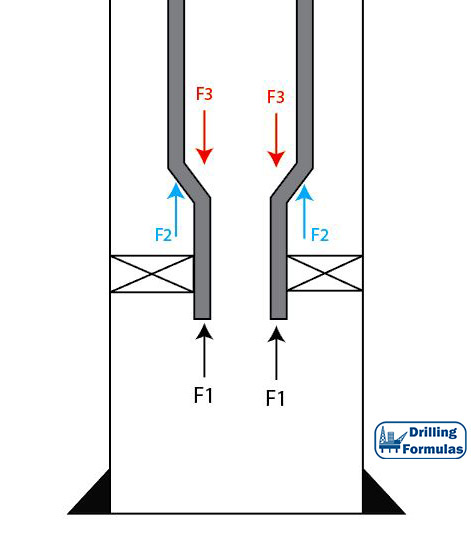Figure 1 – Tapered String Configuration

## Buoyancy Effect on Weight of Tubular Submersed in FluidWhen a tubular submerses into drilling fluid or completion fluid, it will affect how the force distribution works on the tubular. This article will describe how buoyancy will affect tubular weight and a location of neutral axial load (zero axial load).

Weight of Tubing in Air

When tubing is hung in the air, the string weight is equal to linear weight per foot multiplied by the total length of the string. The maximum tensile load is on surface and the zero axial load point is at the bottom.

Tubing Detail

• 5” Tubing
• Weight per length = 17.7 lb/ft
• Total Length = 10,000 ft

Total weight = 17.7 x 10,000 = 177,000 lb

Weight at the bottom of tubing is 0 and weight on top is 177,000 lb in tensile.

Figure 1 demonstrates force distribution of tubing hanging in air.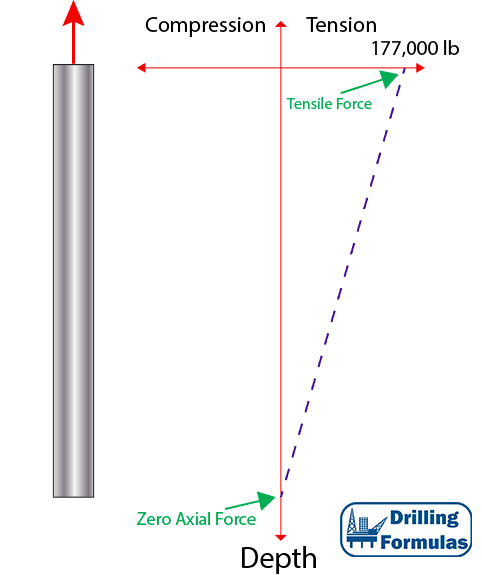Figure 1 – Weight of Tubing in Air

Weight of Tubing When Submersed in Fluid Continue reading